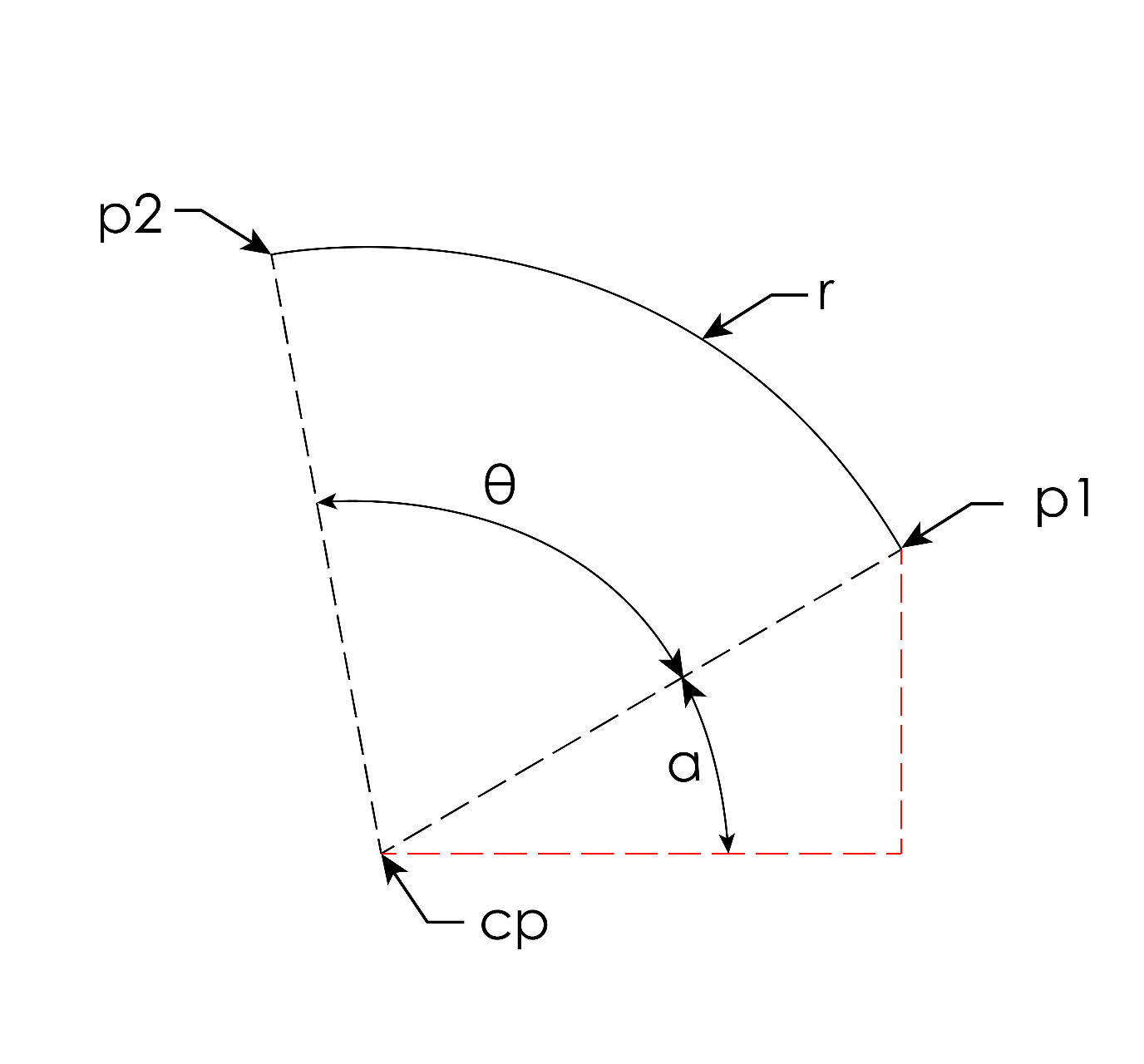Yesenia Obrien

2022-07-14

I have a circle with an arc beginning at point (x,y). The radius is r, the arc angle(w/ respect to center) is $\theta$. How do I calculate the end point of the arc (a,b) ?
I know that the arc-length=radius*(arc angle)
I can't seem to find an easy way to solve this, I think the way to go is with parametric equations but I'm not sure.Sophia Mcdowell

Expert

One way is to calculate the angle to the first point
$\alpha =\mathrm{arctan}\left(\frac{p1.y-cp.y}{p1.x-cp.x}\right)$
$p2.x=cp.x+r\ast cos\left(\alpha +\theta \right)$
$p2.y=cp.y+r\ast sin\left(\alpha +\theta \right)$Do you have a similar question?